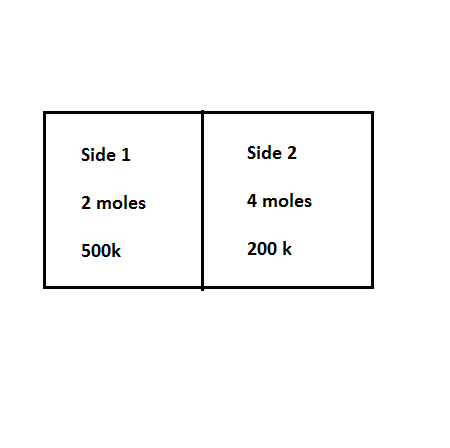A composite system consists of an insulated partitioned volume, shown in Figure 4.9. Side 1...

Question:

A composite system consists of an insulated partitioned volume, shown in Figure 4.9. Side 1 contains 2 mol of a monatomic ideal gas and Side 2 contains 4 moles of the same monatomic ideal gas. Initially, the two sides are separated by a fully insulated partition (adiabatic wall) and the temperatures are 500K and 200K on sides 1 and 2 respectively. At a particular time replacing the partition by a conducting frictionless piston. Initially V1=10L and V2=5L. Let T12 be the common temperature at which equilibrium is reached and P12 the common pressure. a.) Find T12 (Found to be 300K) b.) Find P12, and the new values for V1 and V2. (Found them to be 998kPa, 5L, and 10L respectively) c.) Determine ?S, as in ?S=?S1+?S2.Conservation of Energy:

Energy is a quantity which cannot be created by anyone, or it is not destroyed by anyone. It is only transferred from one place to another place, and it is changed in one form to another form. The energy in every system is conserved after any process. The initial energy is equal to the final energy as per the law of conservation of energy.

Given data:

• The side 1 temperature is: {eq}{T_1} = 500\;{\rm{K}} {/eq}
• The side 2 temperature is: {eq}{T_1} = 200\;{\rm{K}} {/eq}
• The mole in side 1 is: {eq}{n_1} = 2\;{\rm{moles}} {/eq}
• The mole in side 2 is: {eq}{n_2} = 4\;{\rm{moles}} {/eq}

(a)

The expression for the conservation of energy is,

{eq}\begin{align*} {E_i} = {E_f}\\ \dfrac{3}{2}{n_1}R{T_1} + \dfrac{3}{2}{n_2}R{T_2} &= \dfrac{3}{2}{n_1}R{T_{12}} + \dfrac{3}{2}{n_2}R{T_{12}}\\ {T_{12}} &= \dfrac{{{n_1}{T_1} + {n_2}{T_2}}}{{{n_1} + {n_2}}} \end{align*} {/eq}

Substitute the given value in above equation.

{eq}\begin{align*} {T_{12}} &= \dfrac{{\left( 2 \right)\left( {500} \right) + \left( 4 \right)\left( {200} \right)}}{{2 + 4}}\\ {T_{12}} &= 300\;{\rm{K}} \end{align*} {/eq}

Thus, the temperature is {eq}{T_{12}} = 300\;{\rm{K}}. {/eq}

(b)

The expression for the pressure in side 1 is,

{eq}{P_1} = \dfrac{{nR{T_1}}}{{{v_1}}} {/eq}

Substitute the given values in above equation.

{eq}\begin{align*} {P_1} &= \dfrac{{\left( 2 \right)\left( {8.314} \right)500}}{{10 \times {{10}^{ - 3}}}}\\ &= 831.4\;{\rm{kPa}} \end{align*} {/eq}

The expression for the pressure in side 2 is,

{eq}{P_2} = \dfrac{{nR{T_2}}}{{{v_2}}} {/eq}

Substitute the given values in above equation.

{eq}\begin{align*} {P_2}& = \dfrac{{\left( 4 \right)\left( {8.314} \right)200}}{{5 \times {{10}^{ - 3}}}}\\ & = 1330.2\;{\rm{kPa}} \end{align*} {/eq}

The expression for the pressure 12 is,

{eq}{P_{12}} = \dfrac{{{P_1}{V_1} + {P_2}{V_2}}}{{{V_1} + V}} {/eq}

Substitute the given values in above equation.

{eq}\begin{align*} {P_{12}} &= \dfrac{{\left( {831.4} \right)10 + \left( {1330.2} \right)5}}{{10 + 5}}\\ {P_{12}} &\approx 998\;{\rm{kPa}} \end{align*} {/eq}

Thus, {eq}{P_{12}} = 998\;{\rm{kPa}} {/eq}.

The expression for the volume at side 1 is,

{eq}{V_1} = \dfrac{{{P_1}{v_1}{T_{12}}}}{{{T_1}{P_{12}}}} {/eq}

Substitute the given values in above equation.

{eq}\begin{align*} {V_1} &= \dfrac{{\left( {831.4} \right)10\left( {300} \right)}}{{500\left( {998} \right)}}\\ &\approx 5\;{\rm{L}} \end{align*} {/eq}

The expression for the volume at side 2 is,

{eq}{V_2} = \dfrac{{{P_2}{v_2}{T_{12}}}}{{{T_2}{P_{12}}}} {/eq}

Substitute the given values in above equation.

{eq}\begin{align*} {V_2} &= \dfrac{{\left( {1330.2} \right)5\left( {300} \right)}}{{200\left( {998} \right)}}\\ &\approx 10\;{\rm{L}} \end{align*} {/eq}

Thus, the final volumes are {eq}5\;{\rm{L}} {/eq} and {eq}10\;{\rm{L}}. {/eq}

(c)

The expression for the change in entropy of side 1 is,

{eq}\Delta {s_1} = {n_1}c\ln \left( {\dfrac{{{T_{12}}}}{{{T_1}}}} \right) {/eq}

Substitute the given value in above equation.

{eq}\begin{align*} \Delta {s_1} &= 2\left( {12.5} \right)\ln \left( {\dfrac{{300}}{{500}}} \right)\\ &= - 12.77\;{{\rm{J}} {\left/ {\vphantom {{\rm{J}} {\rm{K}}}} \right. } {\rm{K}}} \end{align*} {/eq}

The expression for the change in entropy of side 2 is,

{eq}\Delta {s_2} = {n_2}c\ln \left( {\dfrac{{{T_{12}}}}{{{T_2}}}} \right) {/eq}

Substitute the given value in above equation.

{eq}\begin{align*} \Delta {s_2} &= 4\left( {12.5} \right)\ln \left( {\dfrac{{300}}{{200}}} \right)\\ &= 20.27\;{{\rm{J}} {\left/ {\vphantom {{\rm{J}} {\rm{K}}}} \right. } {\rm{K}}} \end{align*} {/eq}

The change in entropy is,

{eq}\Delta s = \Delta {s_1} + \Delta {s_2} {/eq}

Substitute the given value in above equation.

{eq}\begin{align*} \Delta s &= - 12.77\;{{\rm{J}} {\left/ {\vphantom {{\rm{J}} {\rm{K}}}} \right. } {\rm{K}}} + 20.27\;{{\rm{J}} {\left/ {\vphantom {{\rm{J}} {\rm{K}}}} \right. } {\rm{K}}}\\ \Delta s &= 7.5\;{{\rm{J}} {\left/ {\vphantom {{\rm{J}} {\rm{K}}}} \right. } {\rm{K}}} \end{align*} {/eq}

Thus, {eq}\Delta s = 7.5\;{{\rm{J}} {\left/ {\vphantom {{\rm{J}} {\rm{K}}}} \right. } {\rm{K}}}. {/eq}